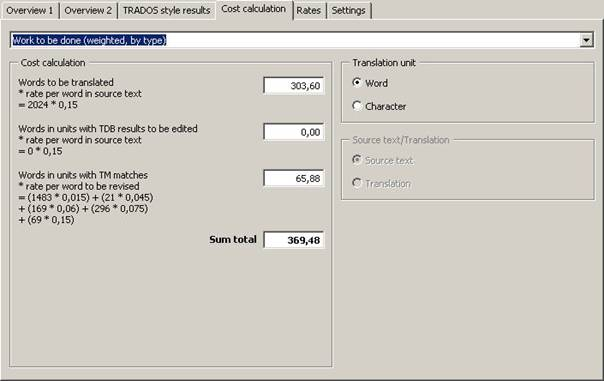##### Cost calculation

This tab contains cost calculations based upon the statistical information retrieved.The Cost calculation frame consists of three elements:

·       You can select the calculation type in the drop-down box located at the top.

·       The calculation results, and some average figures (if appropriate; see below), are shown in the Calculation frame located below.

·       In the Translation unit and Source text/Translation frames, you can select the units used for the calculation (active if appropriate).

Below is a description of the available calculation types:

·       Total per translation unit:

This is the simplest and most widely used calculation for the complete costs of a translation. It has become the worldwide standard for calculating translation costs.

Formula:

Number of words/characters in source text * rate per word/character in source text

Note: Average figures are not available for this calculation type because no meaningful values can be calculated.

·       Work done (per translation unit):

This is a simple way to measure the costs for the work done, based on the standard way to calculate translations costs (see above).

Formula:

Number of translated words/characters in source text/translation * rate per word/character in source text/translation

·       Work done (weighted, by type):

This is a more sophisticated way to calculate translation costs, increasingly used because in some cases the true translation costs are better reflected by this calculation if a translation memory was used for the translation. In MetaTexis, you can now measure the impact of a TM on the translation costs. Even if this impact cannot be measured perfectly, it is possible to make an approximation.

The formula consists of three elements:

Costs for the words/characters in the source text/translation which were translated manually or on the basis of terminology results;

Costs for the words/characters in the source text/translation which were translated by a translation machine.

Costs for the words/characters in the source text/translation that were translated on the basis of TM matches.

Formula:

Number of manually translated words/characters * price per word in source text/translation

+ Number of machine translated words/characters in source text/translation * revision rate per word/character in source text

+ Number of words/characters in source text/translation translated on the basis of TM matches * revision rate per word/character in source text/translation

·       Work to be done (per translation unit):

This is a simple way to measure the costs for the work done, based on the standard way to calculate translations costs (see above).

Formula:

Number of translated words/characters in source text/translation * rate per word/character in source text

+ Number of words/characters in TUs to be edited * rate per word/character in source text

Note: Average figures are not available for this calculation type because no meaningful values can be calculated.

·       Work to be done (weighted, by type):

This calculation supplements the last option. It informs you (or your client) about the costs of the work to be done.

The formula consists of three elements:

Costs for the number of words to be translated

Costs for the number of words in TUs with TDB results

Costs for the number of words in TUs with TM matches

Formula:

Number of words/characters to be translated * rate per word in source text

+ Number of words/characters in TUs with TDB results to be edited * rate per word in source text

+ Number of words/characters in TUs with TM matches to be edited * revision rate per word/character

Note: Average figures are not available for this calculation type because no meaningful values can be calculated.